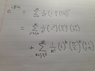# Eigenvectors of exponential matrix (pauli matrix)

• Punctualchappo
In summary, the problem requires finding the eigenvectors and eigenvalues of the matrix exp(iπσx/2), where σx is the x pauli matrix. Using knowledge of the matrix exponential, the problem can be broken down into two sums for even and odd values of n. It is clear that there are only two linearly independent eigenvectors for each sum. The eigenvectors must satisfy the equation exp(iπσx/2)v = Av, where A is the eigenvalue. The eigenvectors of σx are (1,1) and (1,-1).

## Homework Statement

Find the eigenvectors and eigenvalues of exp(iπσx/2) where σx is the x pauli matrix:
10
01

## Homework Equations

I know that σxn = σx for odd n
I also know that σxn is for even n:
01
10

I also know that the exponential of a matrix is defined as Σ(1/n!)xn where the sum runs from n=0 to infinity

## The Attempt at a Solution

Using knowledge of the matrix exponential, I can say that exp(iπσx/2) = Σ(1/n!)(iπσx/2)n. I can then split this into two sums, one for even n and one for odd n. This allows me to take the power off the matrices for easier summing. It's then that I get stuck. I've attached a file showing the two sums because it's easier and clearer to show you this way.[/B]

I'd really appreciate any help that can be given.

#### Attachments

•IMG_3396.JPG
32.5 KB · Views: 899
I've realized that when written as the two sums, it's clear that there are only two linearly independent eigenvectors for each sum. I'm not sure how it works when the i's get multiplied in though. Aren't there infinite eigenvalues to reflect the infinite nature of the sums?

Hi chap, welcome to PF :),

What would an eigenvector of exp(iπσx/2) look like ? What conditions would it have to satisfy ?

And what about eigenvectors of σx itself ?

So the eigenvectors would have to satisfy exp(iπσx/2)v = Av where A is the eigenvalue. I know that eigenvectors of σx are (1,1) and (1,-1)